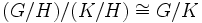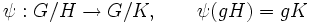Third isomorphism theorem

This article gives the statement, and possibly proof, of a basic fact in group theory.
View a complete list of basic facts in group theory
VIEW FACTS USING THIS: directly | directly or indirectly, upto two steps | directly or indirectly, upto three steps|
View a complete list of isomorphism theorems| Read a survey article about the isomorphism theorems

Statement

Suppose$G$ is a group, and$H$ and$K$ are Normal subgroup (?)s of$G$, such that$H \le K$. Then we have the following natural isomorphism:$(G/H)/(K/H) \cong G/K$

Where the isomorphism sends a coset$Hg$ in$G$ to the coset$Kg$ in$G$.

Note that this statement makes sense at the level of a group isomorphism only when both$H$ and$K$ are normal in$G$. Otherwise, the statement is still true at the level of sets, but we cannot make sense of it as a group isomorphism.

Proof

Given: A group$G$, with normal subgroups$H$ and$K$, such that$H \le K$

To prove:$(G/H)/(K/H) \cong G/K$

Proof: Note first that all the three expressions for quotient groups make sense.$G/H$ and$G/K$ make sense because$H,K$ are normal in$G$. Moreover, since normality satisfies intermediate subgroup condition,$H$ is also normal in$K$.

Next, observe that$K/H$ is a normal subgroup in$G/H$, because normality is image-closed: under the quotient map by$H$, the normal subgroup$K$ of$G$ gets sent to a normal subgroup$K/H$ of$G/H$. Thus, the left side makes sense.

Let's now describe the isomorphism from the left side to the right side:$\psi: G/H \to G/K, \qquad \psi(gH) = gK$

In other words, the map takes a coset of$H$ and gives the corresponding coset of$K$. This is well-defined, because if$h \in H$, then$h \in K$, so$(gh)K = g(hK) = gK$.

Further, the map is a homomorphism. For this, observe that it sends the identity element to the identity element, preserves the group multiplication, and preserves the inverse map.

Further, the map is surjective, because any coset$gK$ occurs as the image of$gH$ under$\psi$.

Finally, we need to determine the kernel of the map. This is given by the set of$gH$ such that$gK = K$. This is precisely those cosets of$H$ that are in$K$, which is the same as the coset space$K/H$. Hence, the kernel of the map is precisely$G/K$.

Thus, the surjective homomorphism$\psi:G/H \to G/K$ has kernel precisely$K/H$. By the first isomorphism theorem, we get:$(G/H)/(K/H) \cong G/K$.